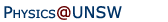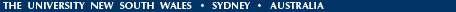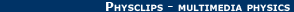Relativity in brief... or in detail..Einstein's theory of special relativity includes electricity and magnetism in a simple, logical extension of the relativity of Galileo and Newton. Its conclusions, including time dilation, length contraction, and E=mc2 have changed profoundly our ideas of time and space, matter and energy.
 The multimedia modules give a brief overview of relativity - they present the main ideas. Inevitably, you will have questions. So the related links (below) give more complete explanations, at levels with () or without mathematics (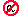). The multimedia modules have animations and film clips and are typically 2Mb. The much smaller HTML versions have only text and images. We are currently replacing the remaining Flash objects (.swf) with .mp4.

Related Links for each module:-

 1. GALILEO - Mechanics and Galilean relativity (Video here, html version here) Related Links Do I need to know this? What will I learn?An introduction to the mechanics of Galileo and NewtonInertial frames and why the laws are the same in the train and on the platformThe original references, and some caveats and conventions.2. MAXWELL - Electricity, magnetism and relativity (Video here, html version here) Related Links A little background to electricity and magnetismElectricity and magnetism in a moving frame: what would you expect?Electricity and magnetism in a moving frame: an electric circuit exampleThe electric and magnetic forces between moving charges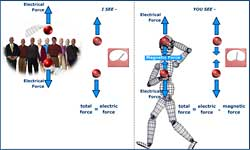3. EINSTEIN - The principle of Special Relativity (Video here, html version here) Related Links Relative to what? What is stationary? The æther?The weirdness - and the logic - of the invariance of the speed of lightWhy Einstein's relativity is an obvious extension of Galileo'sMaxwell's equations: are they really so beautiful that you would dump Newton?But is it true? Is the speed of light really independent of the motion of the observer?More about the Michelson-Morley experiments.4. TIME DILATION - How relativity implies time dilation and length contraction (Video here, html version here) Related Links More about time dilationIs time dilation true? How big are the effects?More about simultaneity, andthe limits to time order reversals.More about length contraction. Is the symmetry of time dilation paradoxical? The twin paradox.Is the symmetry of length contraction paradoxical? The pole paradox.Lorentz transforms, the addition of velocities and spacetime5. E = mc2 - How relativistic mechanics leads to E = mc2 (Video here, html version here) Related Links What do those energy equations mean, and where did they come from?Energy in Newtonian mechanics and in relativityE = mc2 and binding energies in the nucleus (and in molecules.)Why there would be no chemistry without relativityPractical devices using relativityE = mc2: is it true?6. BEYOND RELATIVITY. (Video here, html version here) Related Links The Planck Scale: relativity and quantum mechanics meet (crash into?) gravityPossible variations in the laws of natureOther directions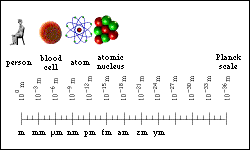Summary (still to be translated from Flash) Written and presented by Joe Wolfe Produced and animated by George HatsidimitrisHome | Summary | Quiz (html version) | Credits School of Physics - The University of New South Wales Sydney, Australia 2052 © School of Physics UNSW 2052 Disclaimer feedback